Ex 4.4

Chapter 4 Class 9 Linear Equations in Two Variables
Serial order wise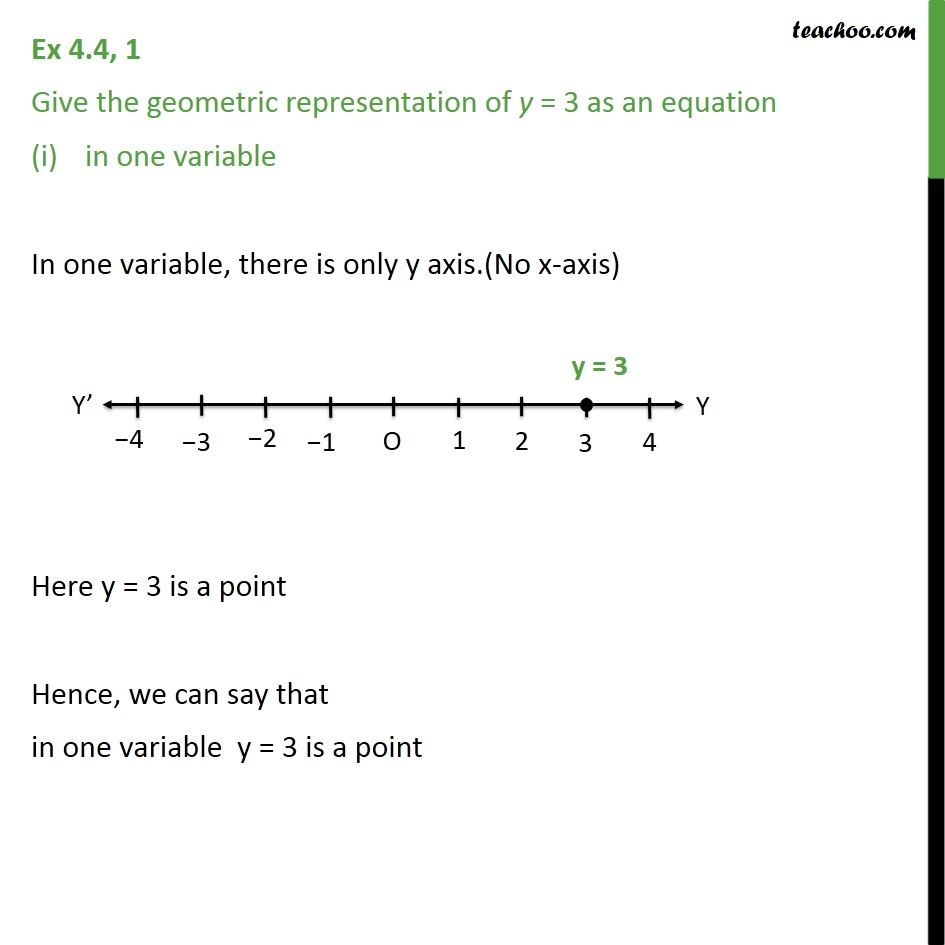This video is only available for Teachoo black users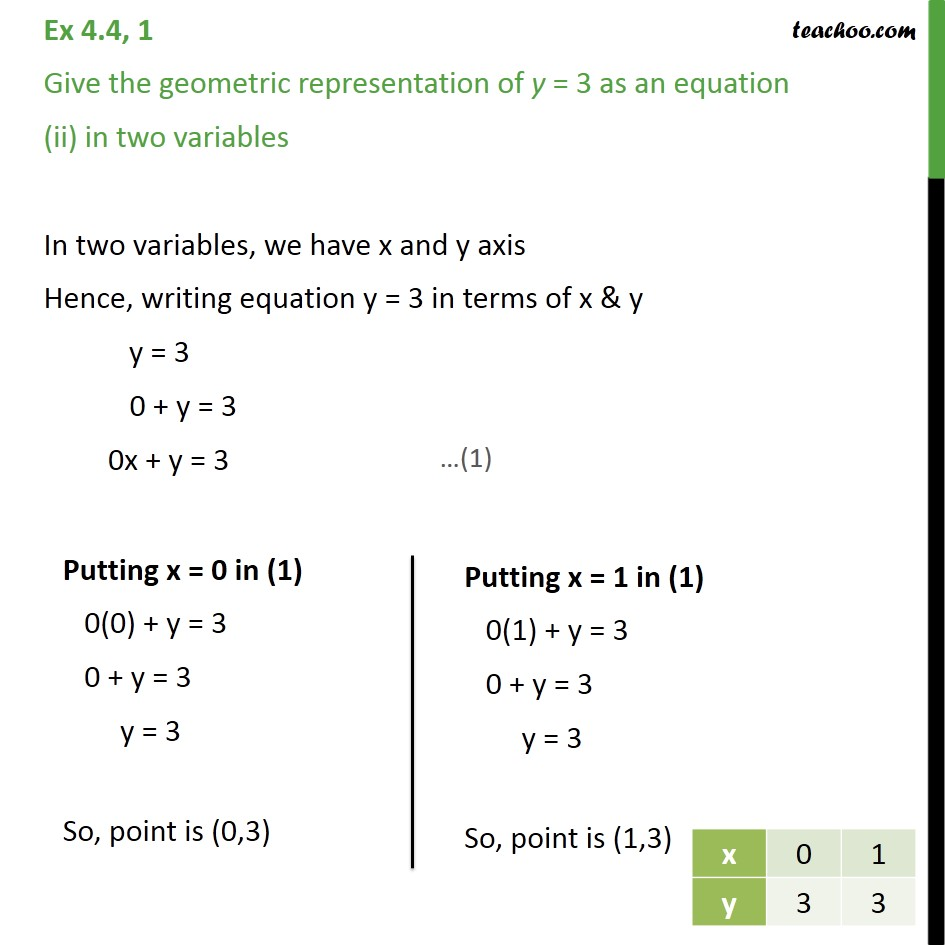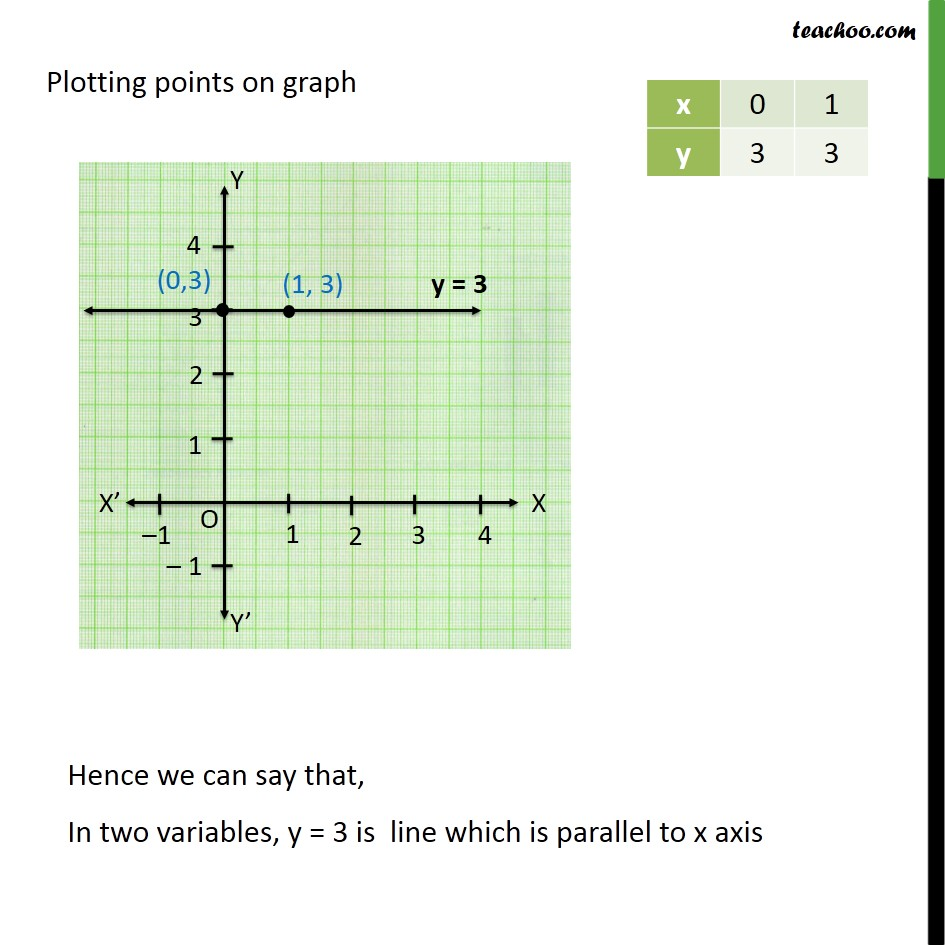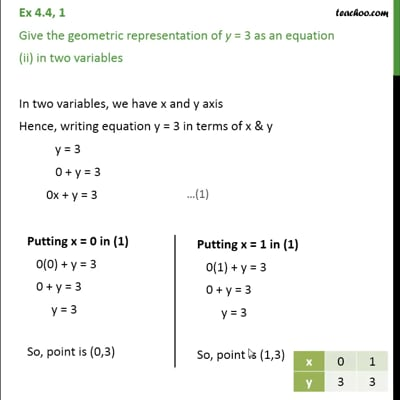This video is only available for Teachoo black users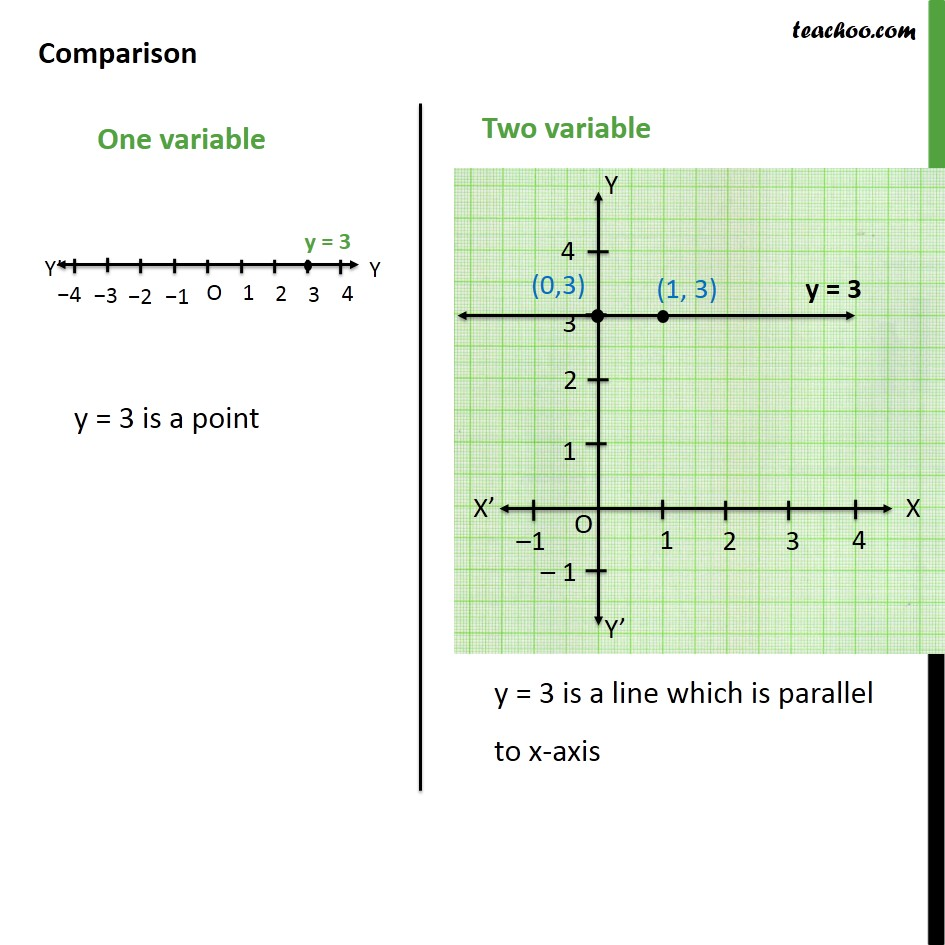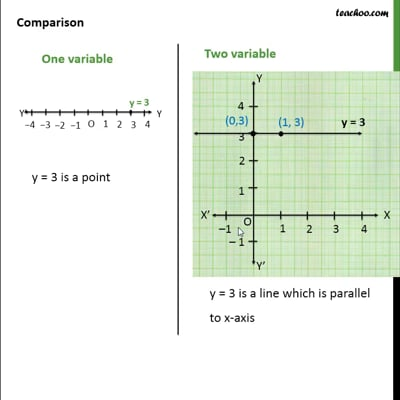This video is only available for Teachoo black users

Maths Crash Course - Live lectures + all videos + Real time Doubt solving!

### Transcript

Ex 4.4, 1 Give the geometric representation of y = 3 as an equation in one variable In one variable, there is only y axis.(No x-axis) Here y = 3 is a point Hence, we can say that in one variable y = 3 is a point Ex 4.4, 1 Give the geometric representation of y = 3 as an equation (ii) in two variables In two variables, we have x and y axis Hence, writing equation y = 3 in terms of x & y y = 3 0 + y = 3 0x + y = 3 Plotting points on graph Comparison